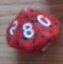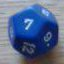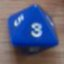Highest/Match 2d10-2d10 open ended roll question

## Highest/Match 2d10-2d10 open ended roll question

 Sun Jan 12 04:46:27 2014   by   Rob TorbenHey, I was hoping you could help me optimize my program here. It seems to be right but it runs very slowly and craps out on some rolls. MECHANICThe mechanic is 2d10 - 2d10 open ending roll where, to reduce the math, you either look for matches, or take the highest number rolled in each dice poolFirst, the dice pool. You have a base positive dice pool of 2d10, and a base negative dice pool of 2d10. Attributes are 0 based, with a +x attribute adding dice to your positive die pool, while a -x attribute adds dice to your negative dice pool.You roll the dice. Any 10 rolled allows you to roll another die and add it to that dice pool. With further tens open ending etc. This works for both dice pools.You then calculate your positive and negative modifiers.First, you look for matches in a dice pool. Your result is equal to the number matched. If you rolled Triples, then the result is equal to 10+ the number matched.4 of a kind gives you a result of 20 the number matched. 5 of a kind, 30+ number matched, etc.If no matches where thrown, you take the highest die rolled as the result of that dice pool. You then subtract the result of negative dice pool from the positive dice pool to get your modifier. Here is the script I came up with\ Match/Highest D10 Dice mechanicP := 3;\Number of Positive Dice (2 + Positive Attribute)N := 2;\Number of Negative Dice (2 + Negative Attribute)\action modifier functionproll := P# (accumulate x:=d10 while x=10);nroll := N# (accumulate x:=d10 while x=10);if (min proll) = (max proll) & (min nroll) = (max nroll) then ( (((count(proll))-2)*10) + (max proll)  ) - ( (((count(nroll))-2)*10) + (max nroll)  )   else if (min proll) = (max proll) & (min nroll) =/= (max nroll) then ( (((count(proll))-2)*10) + (max proll)  ) - (max nroll)  else if (min proll) =/= (max proll) & (min nroll) = (max nroll) then (max proll) - ( (((count(nroll))-2)*10)+ (max nroll) )else max proll - max nroll Mon Jan 13 18:15:25 2014   by   Torben I don't think your script does exactly as you describe since you only distinguish between the cases where all dice in a pool are equal and when they are not all equal.  So you would not, in a pool of 3 dice, find a match of two dice if the third is different.As for performance, one reason for the performance is that statements of the form x:=e forces calculation of the complete distribution for e, so your two assignments force calculation of a large product space.  To reduce this, you have to make sure proll goes out of scope before calculating nroll.  Since your positive and negative pools are handled the same way, the best way to do this is by defining a function that is called twice:`function pool(n) =  roll := n#(accumulate x:=d10 while x=10);  if (count roll) = (count different roll) \ all different  then max roll  else max (foreach i in roll do ((count i = roll)-2)*10+i)P := 3;N := 2;call pool(P) - call pool(N)`This way, the distribution for roll := n#(...) gets out of scope at the end of the function call and what is returned is a much smaller distribution.  Hence, the product space is built from two smaller distributions.Another way to increase performance (at a small cost in precision) is to reduce the iteration bound.  The default is 12, which allows each d10 to be rerolled up to 12 times (which is extremely unlikely).  Reducing it to a smaller number (such as 6), still allows multiple rerolls but computes much faster. Mon Jan 13 22:44:41 2014   by   Rob TorbenThank you for your response! Yeah, I goofed on my formulation. I had one more wrinkle I wanted to examine. As a way to make the dice slightly less explosive I have my 'Top Ten' Rule.Top Tensinstead of automatically exploding a roll of 10, you instead compare 1s and 10s rolled. If there are more 10s than 1s, then each such 10 rolled explodes. Allowing you to roll and add another die.If you roll and add more than one Top Ten die, then you compare the 1s and 10s rolled in that set to determine top tens. If only 1 die is rolled, any 10s automatically explode.How would I implement this, as another function? Tue Jan 14 16:23:51 2014   by   Torben Yeah, another function seems like the best way to do this.  I'm beginning to worry, though, that your mechanism is going to be hard to explain to human users as well as to Troll.In any case, here is a stab at a Troll definition for doing that:`function pool(n) =  roll := n d10;  ones := count 1 = roll;  tens := count 10 = roll;  if tens > ones then roll U call pool(tens - ones) else rollfunction value(roll) =  if (count roll) = (count different roll) \ all different  then max roll  else max (foreach i in roll do ((count i = roll)-2)*10+i)P := 3;N := 2;call value(call pool(P)) - call value(call pool(N))` Tue Jan 14 20:14:17 2014   by   Rob TorbenThank you for assistance and for providing such a great tool to the community! After some quick examination the limited open-ending rule makes too slight a difference to be worth the complication. Thanks again,Rob